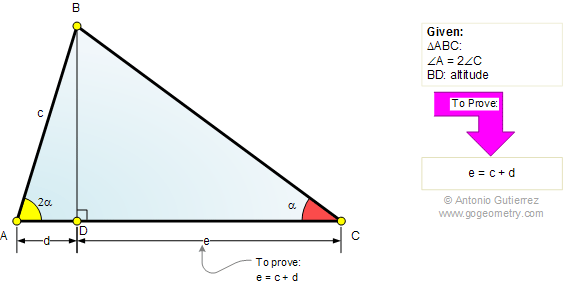Problem 515: Triangle, Double Angle, Altitude, Measure.
Level: High School, College, SAT Prep.

The drawing shows a triangle ABC with the altitude BD. If the angle A is double the angle C, prove that CD=AB+AD.Geometry problem solving is one of the most challenging skills for students to learn. When a problem requires auxiliary construction, the difficulty of the problem increases drastically, perhaps because deciding which construction to make is an ill-structured problem. By “construction,” we mean adding geometric figures (points, lines, planes) to a problem figure that wasn’t mentioned as "given."